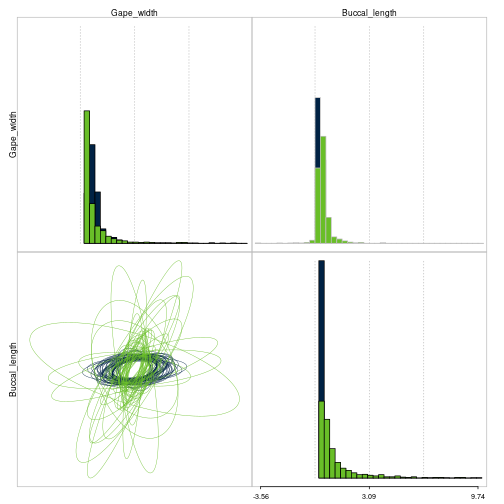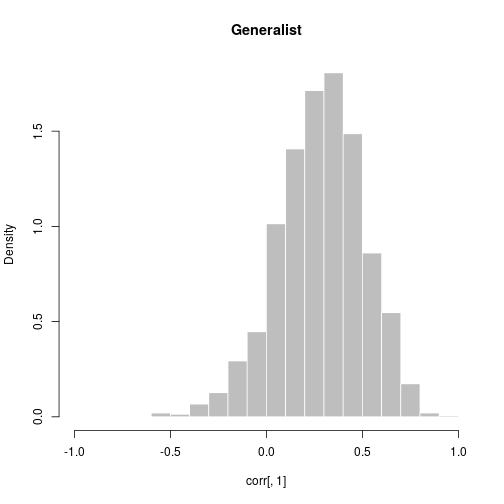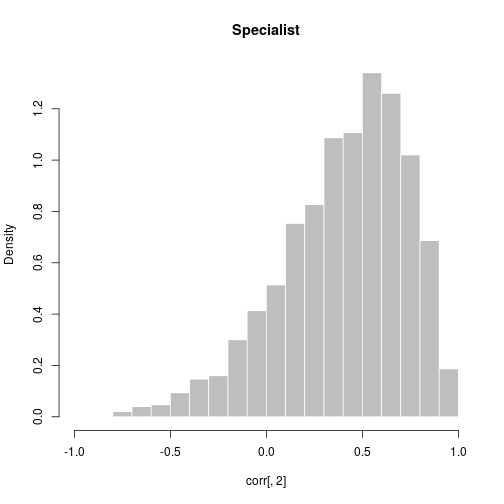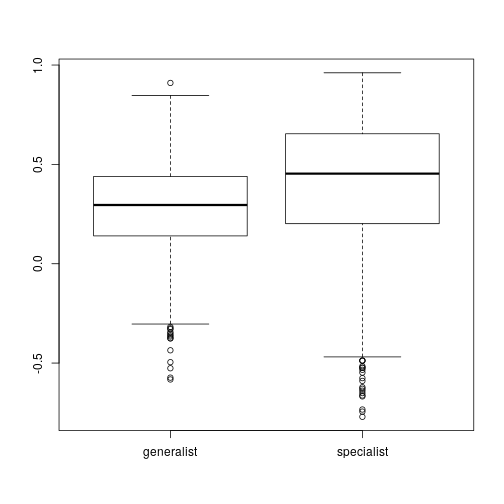# Running a MCMC

Here we will use data on the Centrarchidae fishes to understand how to setup and run a MCMC using the 'ratematrix' package.

There are two main functions on this package: 'ratematrixMCMC' estimates the posterior distribution for the evolutionary rate matrices for one or multiple regimes conditioned on a pool of stochastic maps. 'ratematrixJointMCMC' will perform a joint estimate for both the evolutionary rate matrices and the stochastic maps. So the input for each of the functions differ.

As a general advice, the joint estimation will be better than the estimation conditioned on a fixed pool of stochastic maps. However, there is no problem in using the 'ratematrixMCMC' with a pool of stochastic maps or even a single regime configuration. This option provides flexibility on the way to use the package.

## Input data format

The 'ratematrixJointMCMC' function requires the data for the response trait (continuous), the data for the predictor trait (discrete), and a phylogenetic tree. More information, such as prior distribution, generation number, output name and etc can also be set using the arguments of the function. I strongly recomend reading the help page for the 'ratematrixMCMC' and 'ratematrixJointMCMC' functions before using each.

First we will load the data for the Centrarchidae fishes that accompanies the package.

``````## Load the package
library( ratematrix )
``````
``````## Registered S3 method overwritten by 'geiger':
##   method            from
##   unique.multiPhylo ape
``````
``````## Load the data
data( "centrarchidae" )
## A list with data, phy.map, and pred
names( centrarchidae )
``````
``````##  "data"    "phy.map" "pred"
``````

The data is a matrix with the continuous response traits. This matrix need to have row.names matching the species labels on the phylogeny.

``````resp.data <- centrarchidae\$data
class( resp.data )
``````
``````##  "matrix"
``````
``````head( resp.data )
``````
``````##                     Gape_width Buccal_length
## Lepomis_gibbosus    -0.3468334  -0.030671224
## Lepomis_microlophus -0.3605365   0.016269389
## Lepomis_punctatus   -0.2544218  -0.026716451
## Lepomis_miniatus    -0.3137690   0.007542547
## Lepomis_auritus     -0.5406788  -0.080060653
## Lepomis_marginatus  -0.4471233  -0.152869884
``````

The predictor data is a vector with the information for the rate regimes. The vector need to have names equal to the species labels of the phylogeny.

``````pred.data <- centrarchidae\$pred
names( pred.data )
``````
``````##   "Ambloplites_ariommus"    "Ambloplites_cavifrons"
##   "Ambloplites_rupestris"   "Archoplites_interruptus"
##   "Centrarchus_macropterus" "Enneacanthus_obesus"
##   "Lepomis_auritus"         "Lepomis_cyanellus"
##   "Lepomis_gibbosus"        "Lepomis_gulosus"
##  "Lepomis_humilis"         "Lepomis_macrochirus"
##  "Lepomis_marginatus"      "Lepomis_megalotis"
##  "Lepomis_microlophus"     "Lepomis_miniatus"
##  "Lepomis_punctatus"       "Lepomis_symmetricus"
##  "Micropterus_coosae"      "Micropterus_dolomieu"
##  "Micropterus_floridanus"  "Micropterus_notius"
##  "Micropterus_punctulatus" "Micropterus_salmoides"
##  "Micropterus_treculi"     "Pomoxis_annularis"
##  "Pomoxis_nigromaculatus"
``````
``````## Table show the distribution of the data for the predictor regime.
table( pred.data )
``````
``````## pred.data
## generalist specialist
##         20          7
``````

Finally, we need the phylogeny for the group. The branch lengths are important for this model. It is also important to have an ultrametric phylogeny. Here we will discard the regimes already mapped in this phylogeny in order to perform a joint estimation of the multivariate Brownian motion model and the predictor for the rate regimes.

``````phy <- centrarchidae\$phy.map
## Drop the regimes of the stochastic map.
phy <- mergeSimmap(phy, drop.regimes = TRUE)
``````

Now we have the necessary data to run the analysis.

## Setting up the joint MCMC estimation

For a very quick MCMC analyses we can provide the data, the number of MCMC generations and the directory to run the analyses. You can substitute the argument 'dir' for something more useful, such as dir = “results_mcmc”, to write the results to the folder in the current directory.

``````handle <- ratematrixJointMCMC(data_BM = resp.data, data_Mk = pred.data, phy = phy
, gen = 100000, dir = tempdir())
``````

We can read the results and make a quick plot of the posterior distribution.

``````plotRatematrix(mcmc)
``````
``````## Plotting multiple regimes.
## Table with regimes and colors (names or HEX):
## generalist specialist
##    #002244    #69BE28
``````## Choosing parameters for the MCMC analyses

The previous run used only the default parameters to run the chain. These options will likely work for your data set. But please read the help page for the ratematrixJointMCMC or ratematrixMCMC functions to know what options you are using. Keep in mind that the default prior is a prior that I decided to use. ;) Is it good for you?

## Checking for convergence

The best check for convergence requires to run at least two independent MCMC chains. Let's run a second chain:

``````handle2 <- ratematrixJointMCMC(data_BM = resp.data, data_Mk = pred.data, phy = phy
, gen = 100000, dir = tempdir())
``````

Now we can use the function to check for convergence:

``````Rfactor <- checkConvergence(mcmc, mcmc2)
Rfactor
``````
``````## \$gelman
## \$gelman\$diag_root
## Potential scale reduction factors:
##
##               Point est. Upper C.I.
## Gape_width          1.04       1.15
## Buccal_length       1.19       1.66
##
##
## \$gelman\$generalist
## Potential scale reduction factors:
##
##     Point est. Upper C.I.
## 1,1       1.00       1.02
## 1,2       1.00       1.00
## 2,1       1.00       1.00
## 2,2       1.01       1.01
##
##
## \$gelman\$specialist
## Potential scale reduction factors:
##
##     Point est. Upper C.I.
## 1,1       1.00       1.00
## 1,2       1.02       1.03
## 2,1       1.02       1.03
## 2,2       1.00       1.01
##
##
##
## \$ess
##          root generalist specialist
## var1 54.21500   635.9865   1270.623
## var2 46.98466   621.1375   1064.527
## var3       NA   621.1375   1064.527
## var4       NA   808.4456   1500.000
``````

The output of this function shows both the result of Gelman and Rubin potential scale reduction factor (Gelman's R) for each variable in the model and the estimated effective sample size (ESS) from the all the MCMC chains combined.

A good convergence is achieved when Gelman's R is close to 1 AND the ESS for all the parameters is large. Note that the 200 ESS threshould often used in phylogenetics is not a clear cut. The ESS is the effective size of the sample. In other words, how many points is enough to have a good estimate of the parameter for your model?

The results above show that we should run the MCMC chains for more time. The estimates for Gelman's R are not far from 1, so it will not take too many more generations to achieve good convergence. Here we will assume the sample is good enough for practical reasons.

## Computing summary statistics from the posterior distribution

The “ratematrix” package has some summary statistics ready to be computed from the posterior distribution. Many more are possible. The next topic will show how to extract the posterior samples from the result of the mcmc.

First, let's merge the result from the independent MCMC chains. This help to use all the available results to compute summary statistics.

``````merged.mcmc <- mergePosterior(mcmc, mcmc2)
``````

### Extract the correlation from the model

We can extract and plot the correlation for the model from the merged MCMC. The output from the “extractCorrelation” function will be a matrix with the pairwise correlations among all traits for each of the regimes in the model.

``````corr <- extractCorrelation(merged.mcmc)
dim( corr ) ## 1500 samples and 2 regimes.
``````
``````##  1500    2
``````
``````head( corr )
``````
``````##      generalist specialist
## [1,]  0.1951192  0.4918088
## [2,] -0.1203415  0.4795060
## [3,]  0.4603486  0.3579457
## [4,]  0.4239701  0.8142855
## [5,]  0.3077855  0.8305229
## [6,]  0.4706612  0.9059016
``````

We can make histograms to show the distribution of correlation among the traits. Note that here we have only two traits and one evolutionary correlation estimate for each regime fitted to the phylogeny.

``````hist(x = corr[,1], xlim = c(-1,1), main = "Generalist", col = "grey"
, border = "white", breaks = 20, freq = FALSE)
````````````hist(x = corr[,2], xlim = c(-1,1), main = "Specialist", col = "grey"
, border = "white", breaks = 20, freq = FALSE)
``````We can see that the correlation estimates for the specialist lineages are a little stronger, but the two groups largely overlap each other:

``````boxplot(x = corr)
``````### Using overlap tests

We can apply tests to check the proportion of overlap between the joint posterior estimate for the evolutionary rate matrix regimes. Here we can test for overlap on the rates of evolutio:

``````testRatematrix(chain = merged.mcmc, par = "rates")
``````
``````## []
## []\$`Regime generalist x specialist`
##       [,1]      [,2]
## [1,] 0.696 0.2293333
``````
``````testRatematrix(chain = merged.mcmc, par = "correlation")
``````
``````## []
## []\$`Regime generalist x specialist`
##      [,1]      [,2]
## [1,]   NA 0.7053333
## [2,]   NA        NA
``````

## Extracting the posterior samples of rate matrices

The MCMC object is a list with the posterior samples for all the parameters in the model. Below I show how to extract the posterior distribution for each of the parameters for the model.

Extract the root values. Each row of the matrix is a sample of the MCMC.

``````names( merged.mcmc )
``````
``````##  "root"   "matrix"
``````
``````head( merged.mcmc\$root )
``````
``````##      Gape_width Buccal_length
## [1,]  -0.210077       -0.0412
## [2,]  -0.165792       -0.0323
## [3,]  -0.111355       -0.0576
## [4,]  -0.180007       -0.0886
## [5,]  -0.267360       -0.0993
## [6,]  -0.230780       -0.0796
``````

Extract the evolutionary rate matrices:

``````names( merged.mcmc\$matrix )
``````
``````##  "generalist" "specialist"
``````
``````class( merged.mcmc\$matrix\$generalist )
``````
``````##  "list"
``````
``````length( merged.mcmc\$matrix\$generalist )
``````
``````##  1500
``````
``````gen.rate <- merged.mcmc\$matrix\$generalist
spec.rate <- merged.mcmc\$matrix\$specialist

## The first element of the list:
gen.rate[]
``````
``````##          [,1]     [,2]
## [1,] 1.340940 0.118994
## [2,] 0.118994 0.277358
``````
``````spec.rate[]
``````
``````##          [,1]     [,2]
## [1,] 0.589539 0.174928
## [2,] 0.174928 0.214592
``````

Note that the posterior for the rate matrices are each a list of matrices. So we can use the “lapply” function to extract quantities from each of the samples from the MCMC:

``````## Extracts the rates for the first trait from the posterior distribution of generalists.
rate.tr1.gen <- sapply(gen.rate, function(x) x[1,1])
summary( rate.tr1.gen )
``````
``````##    Min. 1st Qu.  Median    Mean 3rd Qu.    Max.
##  0.0000  0.3463  0.5035  0.6180  0.7695  3.4433
``````

Done!